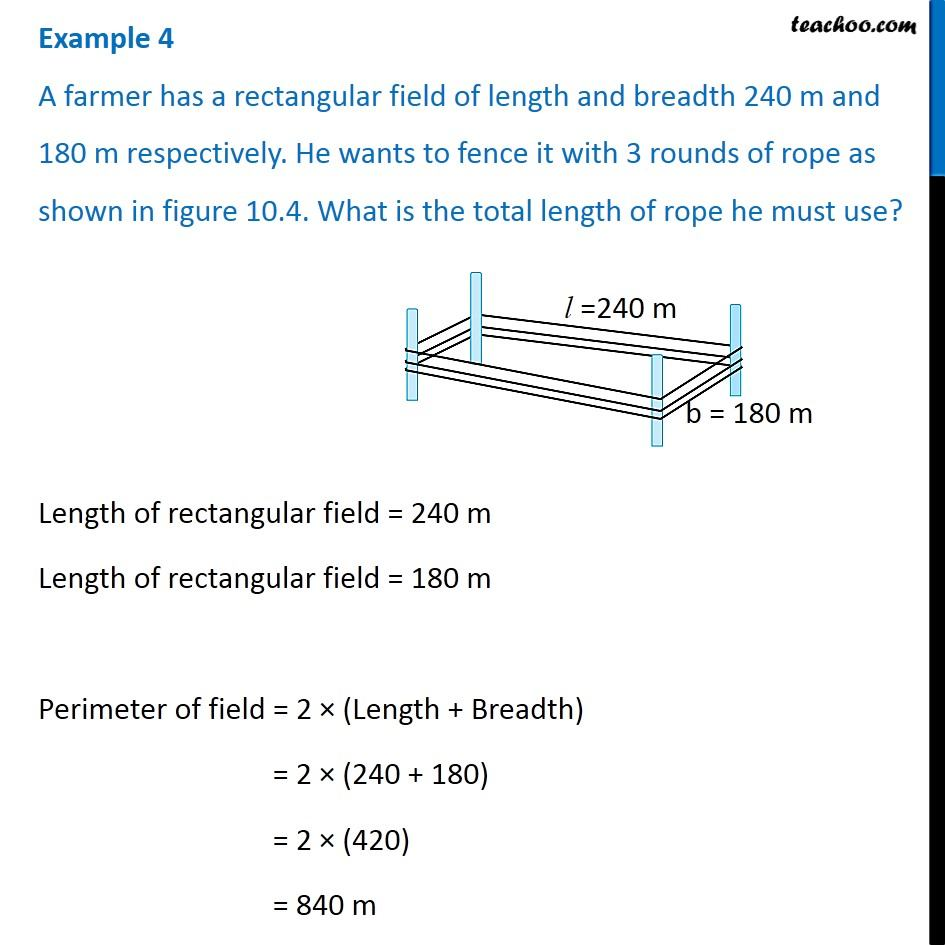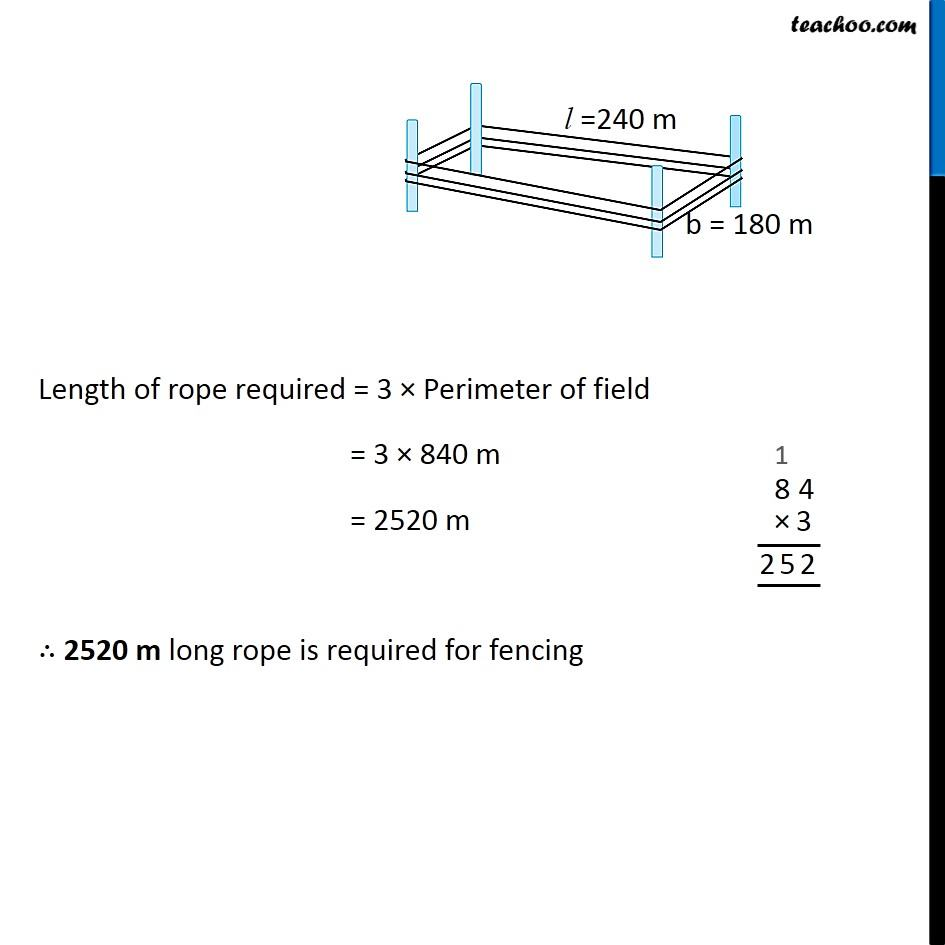Perimeter of Rectangle

Chapter 10 Class 6 Mensuration
Concept wiseGet live Maths 1-on-1 Classs - Class 6 to 12

### Transcript

Example 4 A farmer has a rectangular field of length and breadth 240 m and 180 m respectively. He wants to fence it with 3 rounds of rope as shown in figure 10.4. What is the total length of rope he must use? Length of rectangular field = 240 m Length of rectangular field = 180 m Perimeter of field = 2 × (Length + Breadth) = 2 × (240 + 180) = 2 × (420) = 840 m Length of rope required = 3 × Perimeter of field = 3 × 840 m = 2520 m ∴ 2520 m long rope is required for fencing